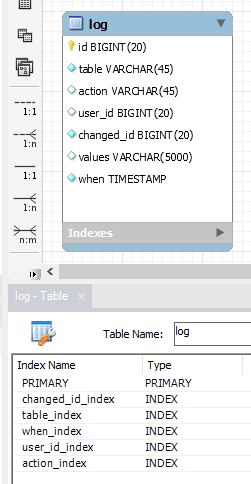# How to construct orthogonal "start_date" data structure.

edited March 2020

This "start_date" data structure looks useful:
https://datatables.net/manual/data/orthogonal-data#Predefined-values
but I can't figure out how to construct that JSON structure when retrieving data from my database. (MySQL with PHP).
Any suggestions welcome.

EDIT: should have mentioned using DT Editor.

edited March 2020

Hey tangerine,

this should work (hopefully):

``````Editor::inst( \$db, 'yourTable' )
->field(
Field::inst( 'yourTable.name        AS name' ),
Field::inst( 'yourTable.position    AS position' ),
Field::inst( 'yourTable.start_date  AS start_date.display' )
->getFormatter( function ( \$val, \$data, \$opts ) {
\$date = new DateTime(\$val);
return \$date->format("d/m/y"); //whatever format you like
} ),
Field::inst( 'yourTable.start_date  AS start_date.timestamp' )
->getFormatter( function ( \$val, \$data, \$opts ) {
\$date = new DateTime(\$val);
return \$date ->format('Y-m-d H:i:s'); //whatever format you like
} ),
Field::inst( 'yourTable.office      AS office' )
)
``````

Since you have helped me generously on multiple occasions: Here is some code that does aliasing of data base columns extensively - and it works! This is about preparing a log for display. The log values are written as a json-string and saved in the log table as per @allan's blog here:
https://datatables.net/blog/2015-10-02#Logging

My challenge was:
- How to read one single field that contains json_encoded values and prepare its content for display as the original (multiple) individual fields?
- How to do this if the log format has changed over time?
- How to do this if two different log formats are used because once the log is written based on DT Editor and once based on proprietary SQL data manipulations?
- How to apply "SELECT DISTINCT" on the result data?
- How to order the result in a way that will be suitable for excel exporting while using a different sort order at the front end display?

This is all being addressed in this code:

``````Editor::inst( \$db, 'log' )
->field(
Field::inst( 'log.id' )->set( false ),
Field::inst( 'log.user_id AS log.changer' )->set( false )
->getFormatter( function(\$val, \$data, \$opts) {
}),
Field::inst( 'log.values AS affected_user' )->set( false )
->getFormatter( function(\$val, \$data, \$opts) {
\$row = json_decode(\$val, true);
if ( isset( \$row["userId"]) ) {
}
if ( isset( \$row["affected_user"]) ) {
}
return '';
}),
Field::inst( 'log.action' )->set( false ),
Field::inst( 'log.values AS log.user_role' )->set( false )
->getFormatter( function(\$val, \$data, \$opts) {
\$row = json_decode(\$val, true);
if ( isset( \$row["ctr_govdept_has_user"]["role"]) ) {
return \$row["ctr_govdept_has_user"]["role"];
}
if ( isset( \$row["role"]) ) {
return \$row["role"];
}
return '';
}),
Field::inst( 'log.values AS user_dept' )->set( false )
->getFormatter( function(\$val, \$data, \$opts) {
\$row = json_decode(\$val, true);
\$govNameStr = '';
if ( isset( \$row["gov"])         &&
isset( \$row["ctr_govdept"]["dept_name"] )  ) {
foreach (\$row["gov"] as \$gn) {
\$govNameStr .= ( \$gn["govName"] . ', ' );
}
\$govNameStr = substr(\$govNameStr, 0, -2);
return \$row["ctr_govdept"]["dept_name"] . ' (' .
\$govNameStr . ')';
}
if ( isset( \$row["ctr_govdept_id"]) &&
isset( \$row["dept_name"])          ) {
\$govArray = getFormatterGovArray(\$row["ctr_govdept_id"]);
foreach (\$govArray as \$gn) {
\$govNameStr .= ( \$gn["govName"] . ', ' );
}
if ( \$govNameStr > '' ) { //we still found the gov
\$govNameStr = substr(\$govNameStr, 0, -2);
return \$row["dept_name"] . ' (' .  \$govNameStr . ')';
}
return \$row["dept_name"];
}
return '';
}),
Field::inst( 'log.values AS dept_id' )->set( false )
->getFormatter( function(\$val, \$data, \$opts) {
\$row = json_decode(\$val, true);
if ( isset( \$row["ctr_govdept_has_user"]["ctr_govdept_id"] ) ) {
return \$row["ctr_govdept_has_user"]["ctr_govdept_id"];
}
if ( isset( \$row["ctr_govdept_id"] ) ) {
return \$row["ctr_govdept_id"];
}
return '';
}),
Field::inst( 'log.when as update_time' )->set( false )
)
->on( 'postGet', function ( \$e, &\$data, \$id ){
\$data = array_values(array_unique(\$data, SORT_REGULAR));
//  do this at the front end in order to make sure boolean values don't get converted
//           for ( \$i=0; \$i < count(\$data); \$i++ ) {
//                foreach ( \$data[\$i] as \$key => \$val ) {
//                    if ( is_numeric( \$val ) ) {
//                        \$data[\$i][\$key] = '#_' . \$data[\$i][\$key];
//                    }
//                }
//            }
array_multisort( array_column(\$data, "affected_user"), SORT_ASC,
array_column(\$data, "dept_id"), SORT_ASC,
array_column(\$data, "update_time"), SORT_ASC,
\$data );
})
->process(\$_POST)
->json();
``````
• That is some epic code @rf1234 - thanks for sharing!

Allan

• Wow! @rf1234, now that's what I call a response! Much appreciated.
This will take me a while to digest. Meanwhile - well, wow!

Thanks guys! Always a pleasure for me to help because I know what you are doing for the DT community. Outstanding.

I didn't like doing this for each and every field:

``````\$row = json_decode(\$val, true);
``````

But it turned out to be more efficient than the solution below that avoids doing this for every field: It simply ran a lot faster than the code below (shortened).

The use of indexes in the log table was of critical importance for performance! Don't hesitate to index pretty much every field (except the "values" field that contains the JSON). It (theoretically) slows down log writing but it certainly helps a lot reading the log. (I deleted all of the where clauses and joins in the code examples. Hence it isn't quite obvious why indexes are really required ... but they are ...)``````Editor::inst( \$db, 'log' )
->field(
Field::inst( 'log.id' )->set( false ),
Field::inst( 'log.user_id' )->set( false ), //changer_id
Field::inst( 'log.values' )->set( false ),
Field::inst( 'log.user_id AS log.changer' )->set( false ),
Field::inst( 'log.action' )->set( false ),
Field::inst( 'log.when as log.update_time' )->set( false ),
Field::inst( 'log.user_id AS log.serial' )->set( false ),
Field::inst( 'log.user_id AS log.ctr_name' )->set( false ),
Field::inst( 'log.user_id AS log.ctr_partner' )->set( false )
)
->on( 'postGet', function ( \$e, &\$data, \$id ){
\$data = array_values(array_unique(\$data, SORT_REGULAR));

for (\$i=0; \$i < count(\$data); \$i++) {

\$row = json_decode(\$data[\$i]["log"]["values"], true);
//                unset(\$data[\$i]["log"]["values"]); //doesn't have to be passed to the client side
\$data[\$i]["log"]["values"] = '';

\$data[\$i]["log"]["changer"] = '';
if ( isset(\$row["ctr"]["updaterName"]) ) {
\$data[\$i]["log"]["changer"] = \$row["ctr"]["updaterName"];
}

\$data[\$i]["log"]["serial"] = '';
if ( isset(\$row["ctr"]["serial"]) ) {
\$data[\$i]["log"]["serial"] = \$row["ctr"]["serial"];
}

\$data[\$i]["log"]["ctr_name"] = '';
if ( isset(\$row["ctr"]["ctr_name"]) ) {
\$data[\$i]["log"]["ctr_name"] = \$row["ctr"]["ctr_name"];
}

\$data[\$i]["log"]["ctr_partner"] = '';
if ( isset(\$row["ctr"]["ctr_partner"]) ) {
\$data[\$i]["log"]["ctr_partner"] = \$row["ctr"]["ctr_partner"];
}
}
})
->process(\$_POST)
->json();
``````
This discussion has been closed.